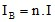# Describing the sources

## Introduction

It is possible to distinguish the following different types of sources:

• permanent magnets
• a DC electric current (uniform distribution of current density)
• an imposed magnetic field (3D applications)
• an imposed magnetic flux (2D applications)

The modeling of these different source types with Flux is presented in the following paragraphs.

## Permanent magnets

The permanent magnets are described using the magnetic material regions as previously presented (see § Describing material media ).

The characteristics of a permanent magnet, remanent induction and the magnetization direction, are introduced during the material definition.

## Electric currents

The electric currents are continuous (time independent) and uniformly distributed, without any space variation.

The electric current sources are described:

## Expression of the current in a coil

The value of the current in a wire (or turn) of the coil (I) is set by means of an electric component (of stranded conductor type) associated to the coil.

The value of the current passing through the cross-section of the coil (IB) can be written:, where:

• n is the number of coil turns
• I is the current through a turn## Role of an electric component

An electric component of stranded conductor type allows one:

• on the one hand, to regroup the coils that are passed through by the same current (meshed or non-meshed coils)
• on the other hand, to define the value of the current

Regrouping of the coils:

An example about coils regrouping is presented below. The current in each coil is the current of the electric component (B1). The coils are series connected.Expression of the current:

There are two ways to define the value of the current in an electric component of stranded conductor type:

• either by directly imposing a value of the current
• or relating the component to an external electric circuit (not valid for a Magnetostatic application)

## Imposed magnetic field (3D)

It is possible to impose an "additional" magnetic field (H0) as in the study domain. This can be a magnetic field created by a dipole, by the magnetic earth field, or created “far” from the modeled part of the device.

This magnetic field is superimposed on the magnetic field created by other sources (permanent magnet, coils).

It can be:

• the field created by a magnetic dipole
• a magnetic field, uniform or space dependent

## Imposed magnetic flux (2D)

It is possible to impose a magnetic flux (φ0) in a region by means of the regions known as non-material of the type: imposed magnetic flux.

The magnetic flux imposed on a line region (2D) is expressed in Webers. This can be uniform or space dependent.